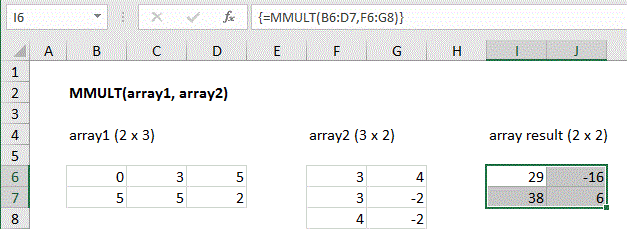## Excel Office

Excel How Tos, Tutorials, Tips & Tricks, Shortcuts

# How to use Excel MMULT Function

This Excel tutorial explains how to use the MMULT function with syntax and examples.

## Excel MMULT function Description

Excel MMULT function returns the matrix product of two arrays. The array result contains the same number of rows as array1 and the same number of columns as array2. If returning multiple results in an array on the worksheet, enter as an array formula with control + shift + enter.

Also See:   Index and match on multiple columns in ExcelExplanation: The MMULT function returns the matrix product of two arrays. The column count of array1 must equal the row count of array 2. The array result will contain the same number of rows as array1 and the same number of columns as array2.  For example, you can multiply a 4 x 2 array by a 2 x 2 array to return a 4 x 2 array result.

Also See:   Count rows that contain specific values in Excel

If returning multiple results in an array on the worksheet, enter as a multi-cell array formula with control + shift + enter.

### Return

The matrix product of two arrays

### Syntax

MMULT (array1, array2)

### Arguments

• array1 – The first array to multiply.
• array2 – The second array to multiply.
Notes:
• Arrays must contain only numbers.
• Columns in array1 must equal the rows in array2.
• Array1 and Array2 can be provided as cell ranges, array constants, or references.
• Formulas that return arrays must be entered as array formulas using control + shift + enter.
• MMULT returns the #VALUE! error if any cells in array1 and array2 are not numbers, or if array1 columns do not equal array2 rows.
Also See:   Count rows with at least n matching values